### python中input（）函数详解

1.input（）函数赋值后数据在python内部的类型。

``````if __name__=="__main__":
a=input()
print(type(a))
b=input()
print(type(b))
c=a+b
print(c)
print(type(c))
``````
• 1
• 2
• 3
• 4
• 5
• 6
• 7
• 8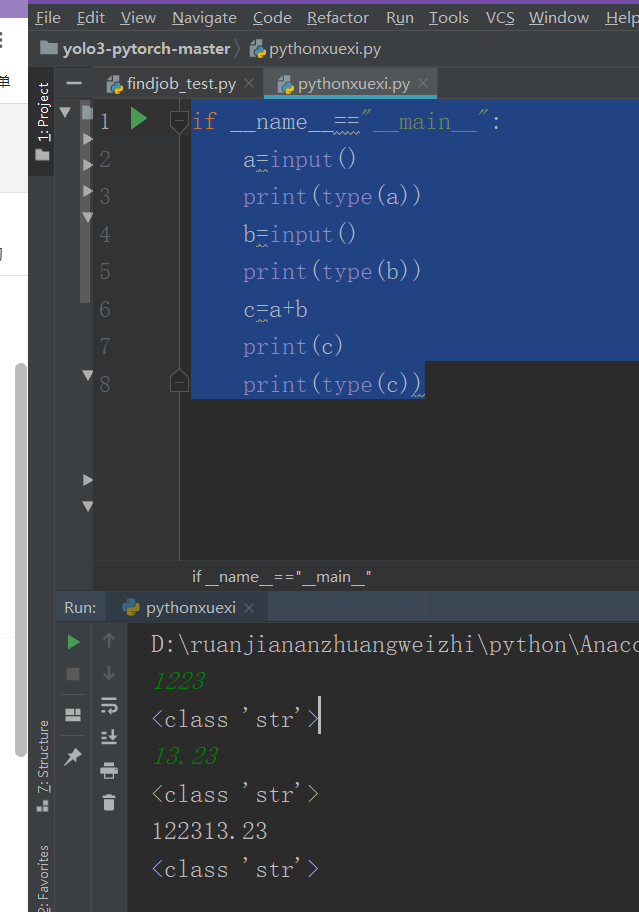2.input（）函数读取数据的转换

``````if __name__=="__main__":
a=int(input())
print(type(a))
b=float(input())
print(type(b))
c=a+b
print(c)
print(type(c))
``````
• 1
• 2
• 3
• 4
• 5
• 6
• 7
• 8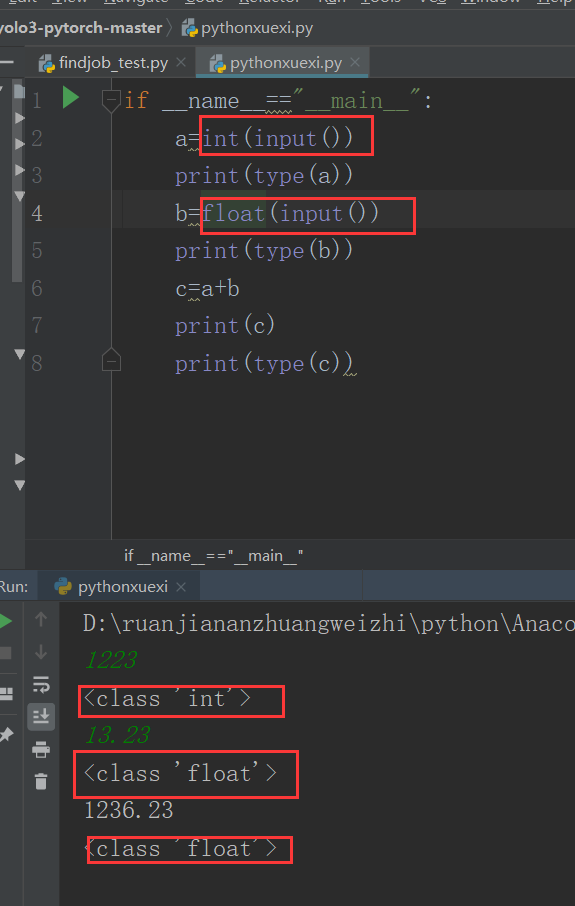3.input（）函数的交互性

``````if __name__=="__main__":
a=int(input())
print(type(a))
b=float(input())
print(type(b))
c=a+b
print(c)
print(type(c))
name=input("请输入您的名字")
print(name)
number=input("请输入"+str(name)+"同学的学号")
``````
• 1
• 2
• 3
• 4
• 5
• 6
• 7
• 8
• 9
• 10
• 11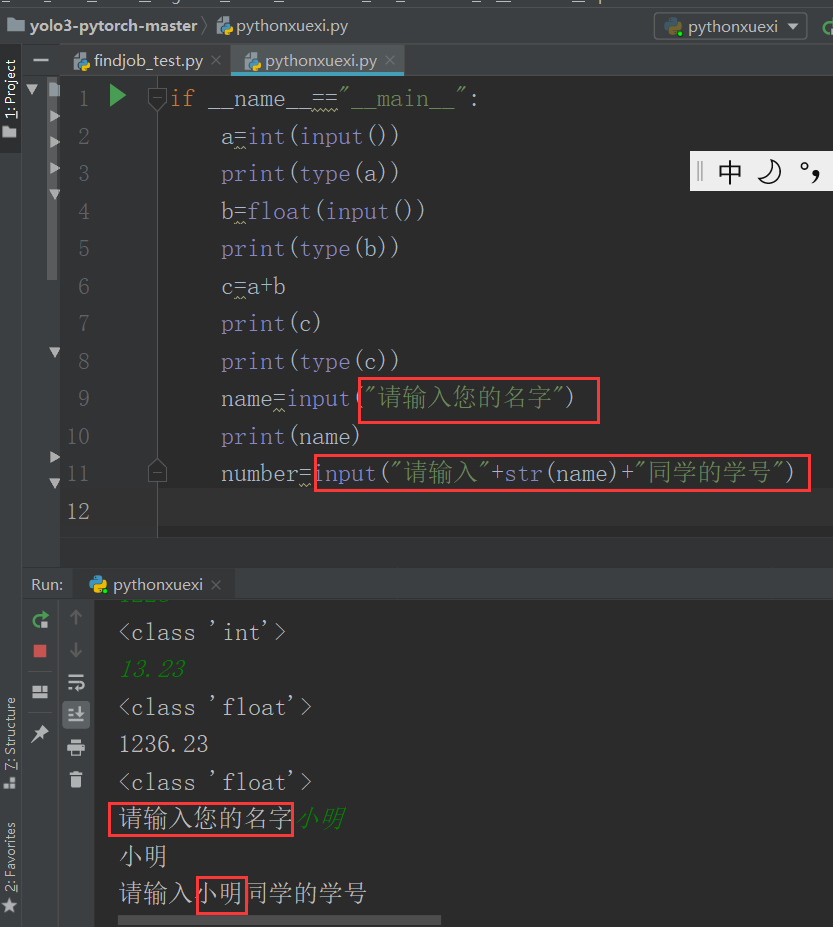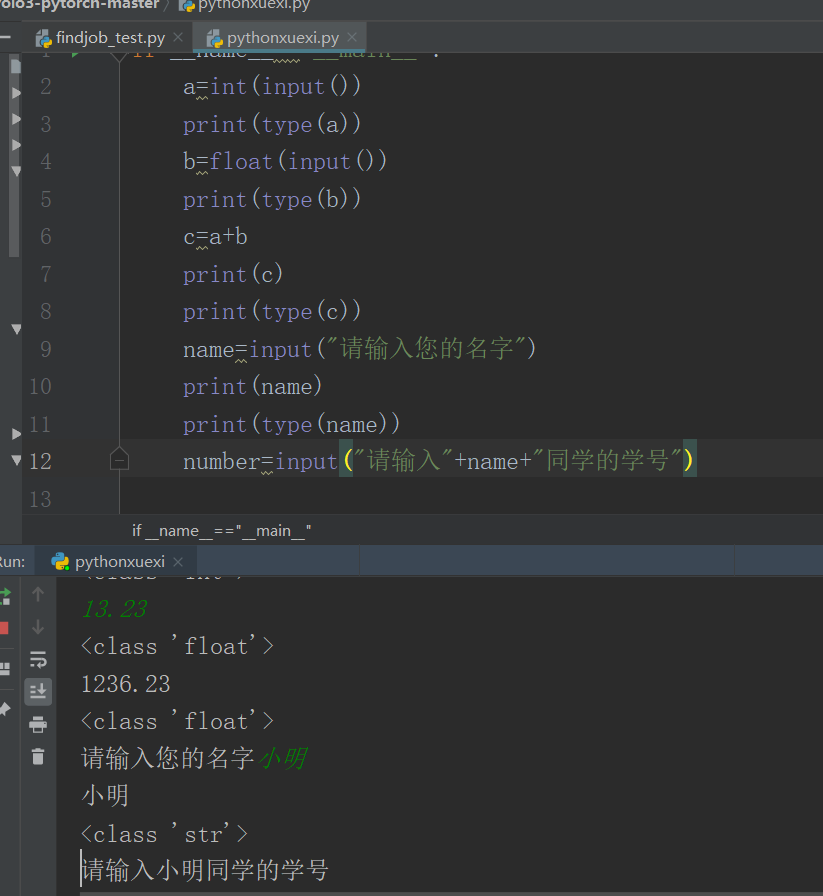4.input（）函数一次读取多个变量
4.1利用split（）函数进行输入的分割，一般默认为空格分割符，也可以自己设置。

``````if __name__=="__main__":
list1=input("请输入一个列表：\n").split(" ")
print(type(list1))
list2= input("请输入一个列表：\n").split(",")
print(type(list2))
print(type(list1))
``````
• 1
• 2
• 3
• 4
• 5
• 6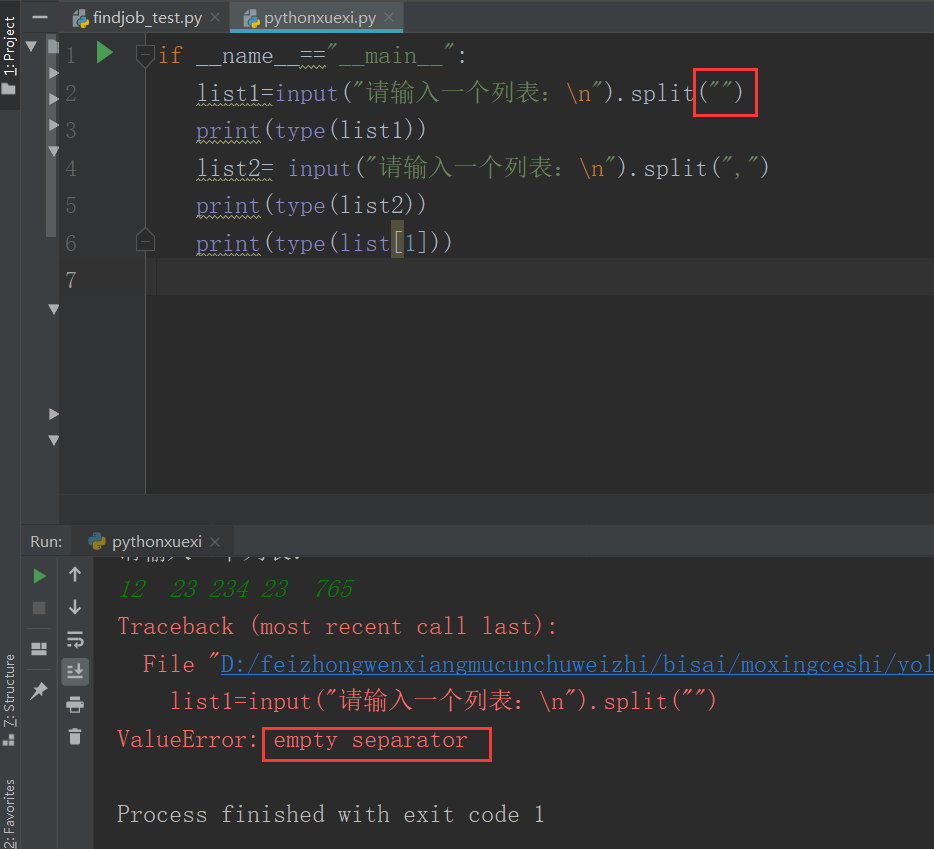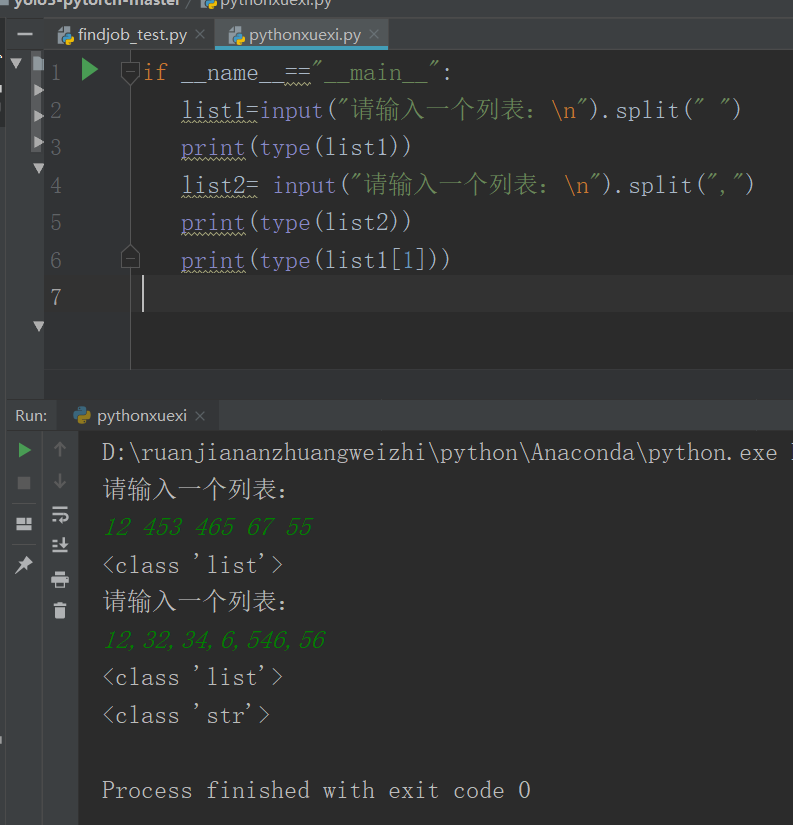4.2input(）函数一次性输入多个变量值

``````if __name__=="__main__":
a,b,c=input("请输入多个变量值：\n").split()
print(a)
print(b)
print(c)
print(type(c))
``````
• 1
• 2
• 3
• 4
• 5
• 6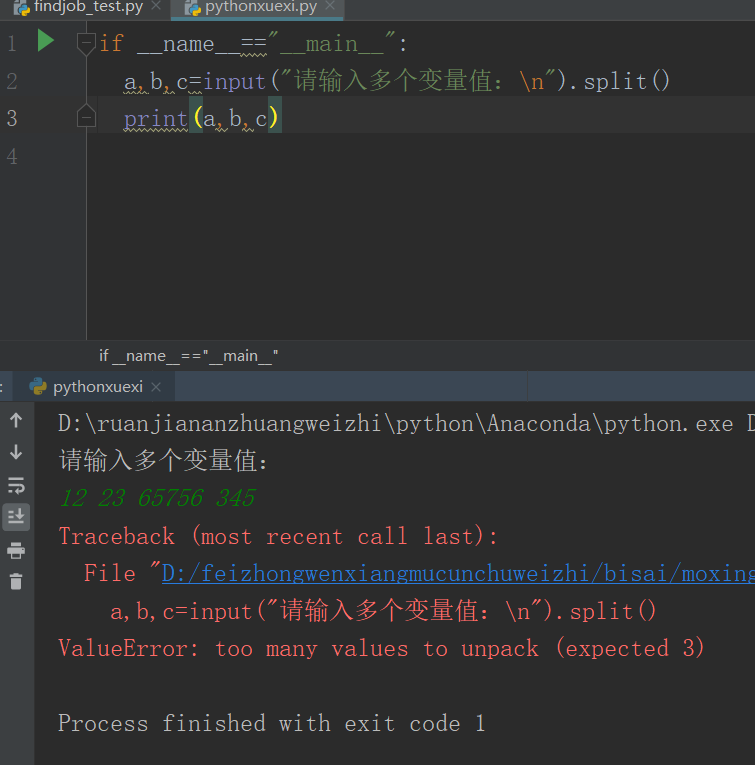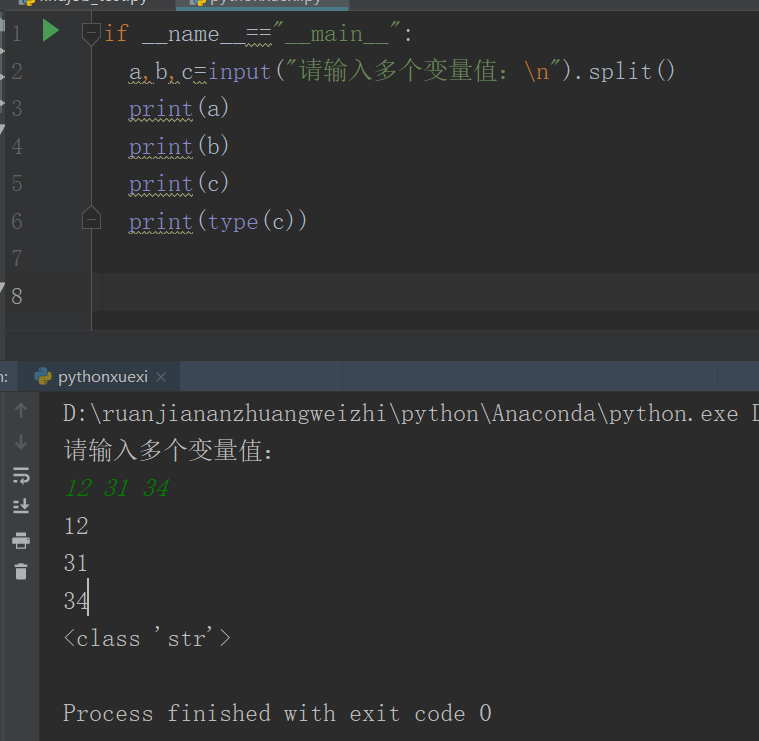4.3将多个变量同时进行类型强制转换
map（）函数用来实现多个输入的强制类型转换，map（）函数接收两个参数，一个是强制转换的类型函数，一个是序列。map（）函数将将强制转换类型的函数依次作用到序列的每个元素，并将结果作为list进行返回。

``````if __name__=="__main__":
a,b,c=map(int,input("请输入多个变量值：\n").split())
d,e,f=[1,2,3]
print(a)
print(type(a))
print(d)
print(e)
``````
• 1
• 2
• 3
• 4
• 5
• 6
• 7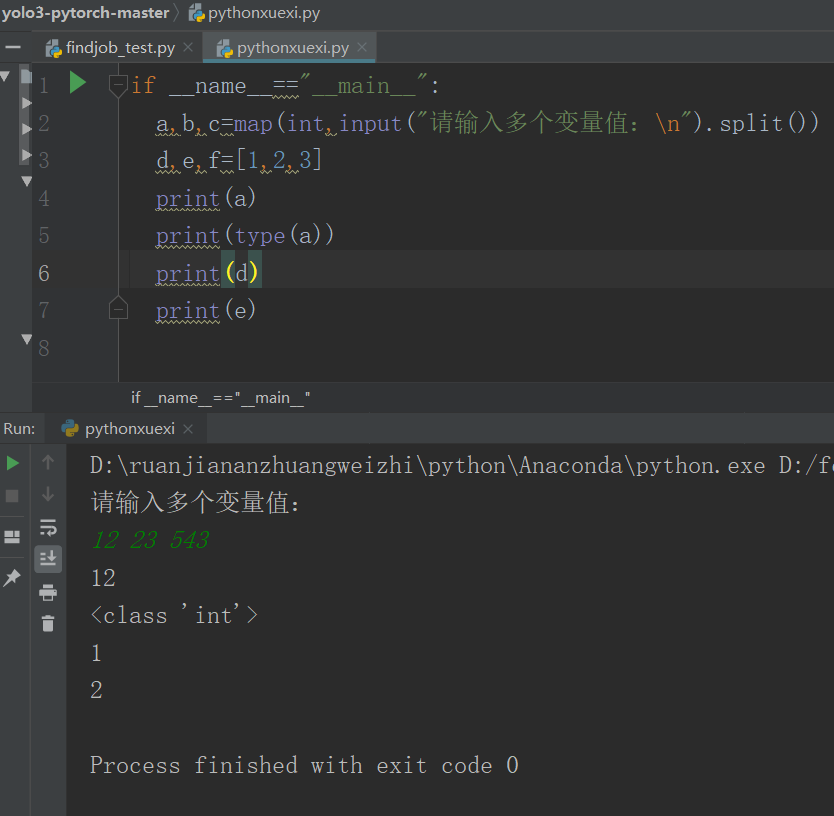（1）列表可以直接赋值给多个变量。
（2）split（）函数的返回值是列表。
（3）map（）函数的返回值是对象。